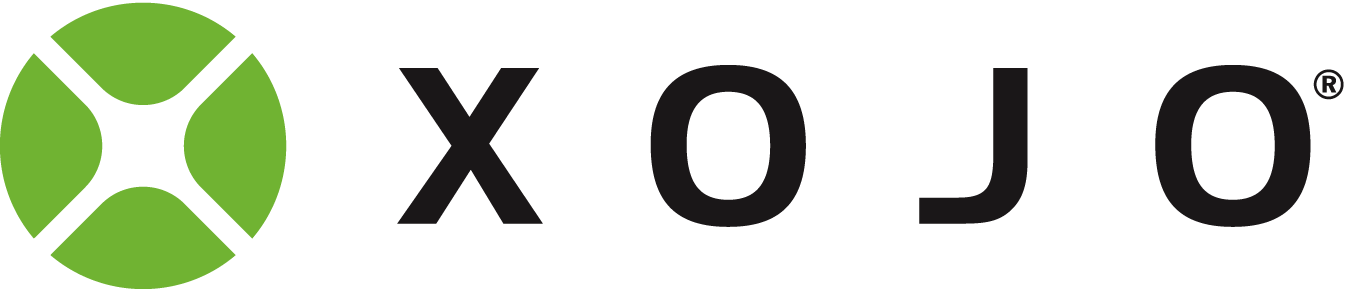# A method is missing from MSOfficeAutomation.rbx - how to workaround?

Am automating Excel with Xojo. It seems that the method “End” in Class ExcelRange is missing. Example: xlsheet.Cells(…).[b]End/b

Any suggestions for a workaround?

Oops! it’s there …

So why doesn’t this compile?
dim lastrow as integer = xlsheet.Cells(100, “A”).End(-4162).Row

[quote=453228:@Mike Linacre]So why doesn’t this compile?
dim lastrow as integer = xlsheet.Cells(100, “A”).End(-4162).Row[/quote]
Whats the error message?

Good morning Mike,

The word ‘End’ is a reserved word in Xojo. I tried the following line of code with an underscore behind End and it works on my machine:

``dim lastrow as integer = xlsheet.Cells(100, "A").End_(-4162).Row``

Here is some example code that returns the correct value of 50 in Column A:

[code]Sub Action() Handles Action
Dim excel as new ExcelApplication
excel.Visible = True

//Fill some rows with data
Dim i as Integer
For i = 1 to 50
excel.Range(“A” + CStr(i)).value =“5”
excel.Range(“B” + CStr(50+i)).value =“6”
Next i

//Show the number of rows
Dim LastRow as Integer
LastRow = Excel.Cells(100, “A”).End_(-4162).Row
MsgBox “The number of rows that have data” + chr(10) + chr(13) +_
"in column A is: " +LastRow.ToText
End Sub
[/code]

Happy to help.Edit: Removed old commented code

Um, that code that causes a misleading result message if the data in the column is not contiguous.

Right after the For/Next loop, I added

``Excel.Range("A77").value = "3"``

which adds the value of 3 in cell A77. The number of rows in column A that contain data is now 51, but your code returns 77. So what you are actually returning is the number of the last row in the column that has data. The code is correct, the msgbox is misleading.

Thanks Dale. Yes, I want the last row in the column that has data. Great! Yes, a global replace fixed this problem everywhere (at least at compile-time). Testing is someway off yetThanks, Eugene and Dale.
Have now a fully operational version in Xojo of the VB6-VBA Excel application. Only one type of VBA instruction is not working:

Purpose: to name a Trendline on an Excel plot.

Original VBA:
xlchart.SeriesCollection(2).Trendlines.Add(Type:=xlPolynomial, order:=2, Forward:=0, Backward:=0, DisplayEquation:=False, DisplayRSquared:=False, Name:=“Upper two-sided 95% Confidence Interval”)

In Xojo, this part works:

This part doesn’t:
xlchart.SeriesCollection(2).Trendlines.Name = “Upper two-sided 95% Confidence Interval”
nor does this :
xlchart.SeriesCollection(2).Trendlines.Name (“Upper two-sided 95% Confidence Interval”)
nor does this:
xlchart.SeriesCollection(2).Trendlines(1).Name = “Upper two-sided 95% Confidence Interval”
nor does this:
xlchart.SeriesCollection(2).Trendlines.Add (xlPolynomial,2,0,0,0,False,False, “Upper two-sided 95% Confidence Interval”)

Any suggestions?

Here you have 0,0,0:

and the original VBA only has 2 (Forward=0, Backward=0). Could that be?

Hi Alberto. Thanks for the reply.

The original VBA does not include the Intercept:= parameter. This is included in MSOfficeAutomation.rbx which says:

Function Add(Type_Param as integer, Order_Param as variant, Period_Param as variant, Forward_Param as variant, Backward_Param as variant, Intercept_Param as variant, DisplayEquation_Param as variant, DisplayRSquared_Param as variant, Name_Param as variant) as ExcelTrendline

And here is ExcelTrendline

Class ExcelTrendline
inherits OLEObject
dim Application as ExcelApplication
dim Backward as integer
dim Border as ExcelBorder
dim Creator as integer
dim DataLabel as ExcelDataLabel
dim DisplayEquation as boolean
dim DisplayRSquared as boolean
dim Forward as integer
dim Index as integer
dim Intercept as double
dim InterceptIsAuto as boolean
dim Name as string
dim NameIsAuto as boolean
dim Order as integer
dim Parent as OLEObject
dim Period as integer
dim Type as integer
Function ClearFormats() as variant
Function Delete() as variant
Function Select_() as variant
End Class

Does this provide any clues?

Hi Mike,

I started a new conversation at: Add Excel trendline Name to answer your new question.Forum for Xojo Programming Language and IDE. Copyright © 2021 Xojo, Inc.